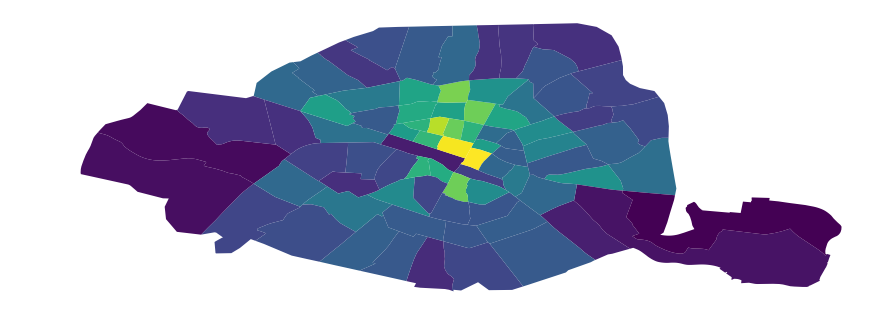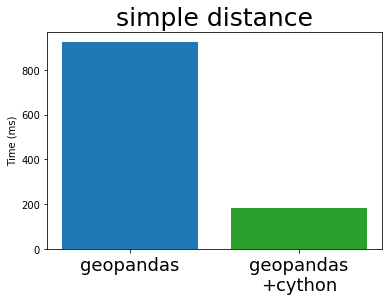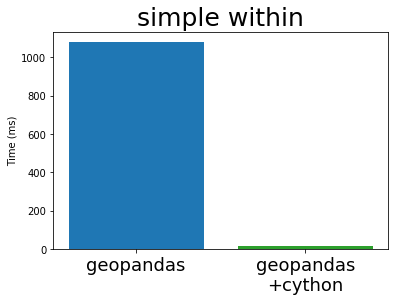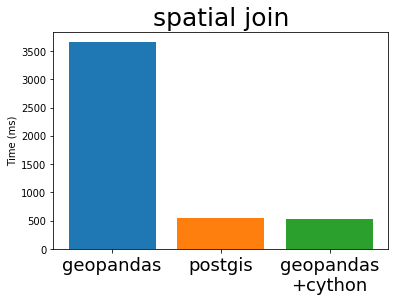# GeoPandas developments: redesign and improved performance using Cython

This work is a collaboration with Matthew Rocklin. This blogpost shows our recent work on the GeoPandas library and is partly based on the EuroSciPy talk (slides) I recently gave on the same topic.

In this blogpost I explain the latest developments in the GeoPandas package. Work is ongoing to vastly improve the performance of the package, by leveraging cython to directly interact with the GEOS library.

Note: what I show here is not yet available in the released version of GeoPandas.

## What is GeoPandas used for?

The goal of GeoPandas is to make working with geospatial data in python easier. GeoPandas extends the pandas data analysis library to work with tables of geospatially annotated data. GeoPandas enables you to easily do operations in python that would otherwise require a spatial database such as PostGIS.

GeoPandas combines the capabilities of pandas with Python's 'geospatial stack' (Shapely to manage geometries like points, linestrings, and polygons; Fiona to handle data import and export, ...). This stack consists of packages that provide intuitive Python wrappers around the OSGeo C/C++ libraries (GDAL/OGR, GEOS, ...) which power virtually every open source geospatial library or application, like PostGIS, QGIS, etc.

### Small demo

For example, we can use GeoPandas to load and plot the Paris districts:

```import geopandas as gpd
```
objectid c_qu l_qu c_ar geometry
0 29 47 Bercy 12 POLYGON ((2.391141037839471 48.82611264577471,...
1 41 1 St-Germain-l'Auxerrois 1 POLYGON ((2.344593389828428 48.85404991486192,...
2 79 76 Combat 19 POLYGON ((2.388343313526396 48.88056667377272,...
3 68 65 Ternes 17 POLYGON ((2.295039618663717 48.87377869547587,...
4 50 10 Enfants-Rouges 3 POLYGON ((2.367101341254551 48.86162755885409,...

Now, let's assume we want to know how many stations of the public bicycle sharing system Vélib (open data) are located in each of the districts. For this, we also load the open data on the bicycle stations, and perform a spatial join with the districts dataframe:

```# load the bicycle stations information
# spatial join: determine in which district each stations is located
stations = gpd.sjoin(stations, df[['l_qu', 'geometry']], op='within')
# count the number of stations per district, and add this information to the
# districts dataframe
counts = stations.groupby('l_qu').size()
df = df.merge(counts.reset_index(name='n_stations'))
# calculate the relative number of bike stations divided by the area
df['n_stations_relative'] = df['n_stations'] / df.geometry.area
```

And now we can make a choropleth plot of the districts of Paris based on the number of bicycle stations:

```ax = df.plot(column='n_stations_relative', figsize=(15, 6))
ax.set_axis_off()
```A larger demo on the basic functionality of GeoPandas, expanding the example above, can be found in this demo notebook from my recent EuroScipy presentation.

## Current design of GeoPandas

Today a GeoDataFrame basically is a pandas dataframe with a special `object`-dtype column that stores Shapely geometries (the 'geometry' column). Shapely geometries are Python objects that provide a Python interface and reference to GEOS Geometry objects in C and provide all the spatial operations:The easy-to-use vectorized operations that GeoPandas provides, such as calculating the distance from every geometry in my dataframe to a certain point:

```df.geometry.distance(some_point)
```

are actually simply small wrappers around Python for loops over shapely calls. So the above method call is roughly implemented as the following:

```def distance(self, other):
result = [geom.distance(other) for geom in self.geometry]
return pd.Series(result)
```

Where each `geom` object in this iteration is an individual Shapely object, and the distance method of this Shapely objects calls into the GEOS library.

This simple design has made GeoPandas a very lightweight and easy-to-develop library, and is possible because GeoPandas can build upon the existing geospatial libraries. But, this simple design has also become a performance bottleneck.

## Performance bottleneck

GeoPandas is slow, which limits its usability for working with larger datasets. This slow performance is because of the current design of GeoPandas shown above: wrapping each geometry (like a point, line, or polygon) with a Shapely object, storing all of those objects in an object-dtype column, and iterating through those objects when performing spatial operations.

The iteration over the individual Shapely geometries is inefficient for two reasons:

1. Iterating in Python is slow relative to iterating through those same objects in C.
2. Calling the geospatial operation on the Shapely Python object gives overhead compared to calling the underlying GEOS C operation directly.

Additionally, the Shapely python objects can take up a significant amount of RAM relative to the GEOS Geometry objects that they wrap.

#### Some timings

Let's explore this bad performance with a small benchmark:

```import geopandas as gpd
from shapely.geometry import Point, Polygon
import random

s = gpd.GeoSeries([Point(random.random(), random.random()) for _ in range(100000)])
polygon = Polygon([(random.random(), random.random()) for _ in range(3)])
```

We have a GeoSeries of 100,000 points and a single polygon. For those objects we calculate the distance from all the points to the polygon, and whether each point lies within the polygon:

```s.distance(polygon)
s.within(polygon)
```

Both of these operations take roughly 1 second to compute.

This may not seem that much, but you have to be aware that geospatial datasets quickly become larger than 100,000 points, and that in more complex analyses those basic operations such as distance computations and set operations often are done many times.

Below, I also compare the performance of GeoPandas to PostGIS, the standard geospatial plugin for the popular PostgreSQL database. While GeoPandas can often be as expressive as PostGIS, we will see that the current version of GeoPandas is also much slower than PostGIS.

## Cythonizing GeoPandas

Fortunately, we can improve upon this by accelerating GeoPandas using Cython to perform the for loops in C and to call the underlying C library GEOS directly.

As an example, the distance function now looks like the following Cython implementation (some liberties taken for brevity):

```cpdef distance(self, other):
cdef int n = self.size
cdef double[:] out = np.empty(n, dtype=np.float64)
cdef GEOSGeometry *left_geom
cdef GEOSGeometry *right_geom = other.__geom__  # a geometry pointer
geometries = self._geometry_array

with nogil:
for idx in xrange(n):
left_geom = <GEOSGeometry *> geometries[idx]
if left_geom != NULL:
out[idx] = GEOSDistance_r(left_geom, some_point.__geom)
else:
out[idx] = NaN
return out
```

For a more complete example how to use Cython to interface directly with GEOS to speed-up shapely operations, see my previous blogpost on this topic.

## New GeoPandas design

To use such cython implementations of the spatial operations, we redesigned the internals of GeoPandas. Instead of using a Pandas `object`-dtype column that holds shapely objects, we instead store a NumPy array of direct pointers to the GEOS objects (images by Matthew Rocklin).

BeforeAfterThis allows us to store data more efficiently, and also allows us to now write our loops over these geometries in C or Cython. When we perform bulk vectorized operations on many GEOS pointers at once, dropping down to Cython or C to write these loops directly provides a significant speedup.

To summarize, we created a numpy array-like object, which we call the `GeometryArray`, to hold geometry objects:

• It only stores integer pointers to the C GEOS geometry objects, without wrapping every geometry in Python shapely objects.
• Operations on the array iterate through those pointers in C / Cython.
• The geometries are wrapped into Python shapely objects on access.

## New benchmarks

When we run the same simple benchmarks from above, we get the following speed-up with the new implementation:This shows a big improvement, depending on the exact operation. For the within operation, we get a 70x speed-up for this example.

#### Comparison to PostGIS

The above benchmarks are timings for basic operations, but let's see what speed-up we see for more complex operations. To this end, I have taken an example of a spatial join from the Boundless PostGIS tutorial (CC BY SA). PostGIS is the standard geospatial plugin for the popular PostgreSQL database, and also uses the GEOS library under the hood.

PostGIS Query

```-- What is the population and racial make-up of the neighborhoods of Manhattan?
SELECT
neighborhoods.name AS neighborhood_name, Sum(census.popn_total) AS population,
100.0 * Sum(census.popn_white) / NULLIF(Sum(census.popn_total),0) AS white_pct,
100.0 * Sum(census.popn_black) / NULLIF(Sum(census.popn_total),0) AS black_pct
FROM nyc_neighborhoods AS neighborhoods
JOIN nyc_census_blocks AS census
ON ST_Intersects(neighborhoods.geom, census.geom)
GROUP BY neighborhoods.name
ORDER BY white_pct DESC;
```

GeoPandas Code

```res = geopandas.sjoin(nyc_neighborhoods, nyc_census_blocks, op='intersects')
res = res.groupby('NAME')[['POPN_TOTAL', 'POPN_WHITE', 'POPN_BLACK']].sum()
res['POPN_BLACK'] = res['POPN_BLACK'] / res['POPN_TOTAL'] * 100
res['POPN_WHITE'] = res['POPN_WHITE'] / res['POPN_TOTAL'] * 100
res.sort_values('POPN_WHITE', ascending=False)
```

The full code example can be found in this notebook. DISCLAIMER: this is just a dummy comparison and no real performance benchmark, and I am not a PostGIS expert.

Timing this spatial join with both PostGIS and the current and cythonized GeoPandas, we get the following result:We can see that the new implementation gives a nice speed-up compared to the current GeoPandas, ánd is now also comparable to the performance of PostGIS. This is not surprising because PostGIS is using the same GEOS library internally.

## Outlook

In this blog post I have shown some promising results, but we have to emphasize this is still a work in progress and there is plenty of work to do. Some of the issues we have to tackle:

• Robust integration with pandas
• Slow data ingestion (currently based on Fiona, wrapper around GDAL/OGR)
• Re-implementing algorithms such as spatial overlays (spatial joins already done in C, directly interfacing GEOS and wrapped in cython to use in GeoPandas)
• Battle testing!

To elaborate on the first item about integration with Pandas: we need for Pandas to track our arrays of GEOS pointers differently from how it tracks a normal integer array. This is both for usability reasons, like we want to render them differently and don't want users to be able to perform numeric operations like sum and mean on these arrays, and also for stability reasons, because we need to track these pointers and release their allocated GEOSGeometry objects from memory at the appropriate times.

Currently, this goal is pursued by creating a new block type, the `GeometryBlock` ('blocks' are the internal building blocks of pandas that hold the data of the different columns). This will require some changes to Pandas itself to enable custom block types (see this issue on the pandas issue tracker).

But next to the challenges, the cythonized GeoPandas version also promises: a speed-up, a memory improvement, and it makes it more feasible to experiment with using GeoPandas with Dask to parallelize or distribute geospatial analyses. See companion blogpost of Matthew Rocklin for such an experiment.

Last, the `GeometryArray` concept might be more broadly useful than just for GeoPandas. If you have such use cases, we would love to hear about that!

### Trying this out!

We would love you to try out the new cythonized GeoPandas version, test it on some real use cases and provide feedback.

Installing the new version currently requires building it from source with Cython. However, if you already have the released version of GeoPandas installed, Cython should be the only additional dependency (the GEOS library to build against should then already be installed). And in that case, the following steps should suffice:

```git clone https://github.com/geopandas/geopandas
git checkout geopandas-cython
make install
```

You can find more information and track future progress on this effort at geopandas/geopandas #473. Also feedback can be posted there, and if you have troubles while trying it out, don't hesitate to ask questions at the geopandas gitter channel.